### Triangles - Test Papers

CBSE Test Paper 01

CH-7 Triangles

1. If all the altitudes from the vertices to the opposite sides of a triangle are equal, then the triangle is

1. Equilateral

2. Isosceles

3. Scalene

4. Right-angled

2.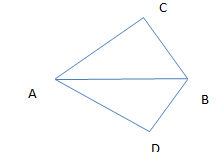In the above quadrilateral ACBD, we have AC = AD and AB bisect the ∠A .Which of the following is true?

1. $\mathrm{△}$ABC $\cong$ $\mathrm{△}$ABD

2. $\mathrm{\angle }$C = $\mathrm{\angle }$D

3. All are true

4. BC = BD

3. AD is the median of the triangle. Which of the following is true?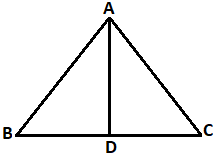1. AC + CD < AB

2. AB + BD < AC

3. AB + BC + AC > AD

4.  AB + BC + AC > 2AD

4. In the adjoining Figure, AB = AC and BD = CD. The ratio $\mathrm{\angle }$ABD : $\mathrm{\angle }$ACD is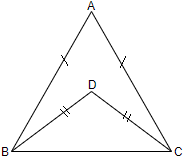1. It is 1 : 1

2. It is 1 : 2

3. It is 2 : 3

4. It is 2 : 1

5. In the adjoining figure, $\mathrm{△}ABC\cong \mathrm{△}ADC$. If $\mathrm{\angle }$BAC = ${30}^{\circ }$and $\mathrm{\angle }$ABC = ${100}^{\circ }$ then $\mathrm{\angle }$ACD is equal to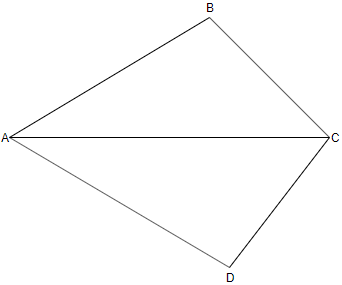1. ${50}^{\circ }$

2. ${80}^{\circ }$

3. ${30}^{\circ }$

4. ${60}^{\circ }$

6. Fill in the blanks:

In a $\mathrm{△}ABC$, AB =  5 cm, AC  = 5 cm and $\mathrm{\angle }B$ equals to ________.

7. Fill in the blanks:

An angle is 4 time its complement, then the measure of the angle is ________.

8. Find the measure of each exterior angle of an equilateral triangle.

9. Compute the value of x of the following given figure: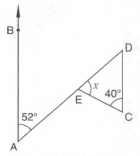10. Prove that $\mathrm{△}$ABC is an isosceles, if Altitude AD bisects ∠BAC.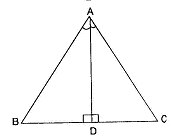11. In figure, if AB $‖$ DC and P is the mid-point of BD, prove that P is also the mid-point of AC.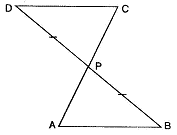12. In Fig, it is given that AE = AD and BD = CE. Prove that $\mathrm{△}AEB\cong \mathrm{△}ADC$.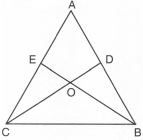13. In figure, AD = AE and D and E are points on BC such that BD = EC. Prove that AB = AC.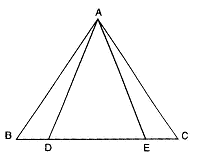14. Show that the difference of any two sides of a triangle is less than the third side.

15. ABCD is quadrilateral such that AB = AD and CB = CD. Prove that AC is the perpendicular bisector of BD.

CBSE Test Paper 01
CH-7 Triangles

Solution

1. (a) Equilateral
Explanation: In an equilateral triangle all  the altitudes,sides, angles, perpendicular bisectors, medians and angular bisectors are equal.
2. (c) All are true
Explanation: In triangle ABC and ABD ,we have
$\mathrm{\angle }$AB = $\mathrm{\angle }$BAD
AB = AB
By SAS ,we have
$\mathrm{\angle }$ABC $\cong$ $\mathrm{\angle }$ABD
Hence, we have BC = BD and $\mathrm{\angle }$C = $\mathrm{\angle }$D.
So,all the given options are true.
3. (d) AB + BC + AC > 2AD
Explanation:
AB + AC + BD + DC > 2AD
Now BD + DC = BC
So, AB + AC + BC > 2AD
4. (a) It is 1 : 1
Explanation:
In $\mathrm{△}$ABC
AB = AC
∴ $\mathrm{\angle }$ABC = $\mathrm{\angle }$ACB(angles opposite to equal sides of a triangle are equal)......1
in ΔDBC,
DB = DC,
∴ $\mathrm{\angle }$DBC = $\mathrm{\angle }$DCB(angles opposite to equal sides of a triangle are equal)......2
subtract 2 from 1
$\mathrm{\angle }$ABC - $\mathrm{\angle }$DBC = $\mathrm{\angle }$ACB - $\mathrm{\angle }$DCB(equals subtracted from equals gives equal)
$\mathrm{\angle }$ABD = $\mathrm{\angle }$ACD
divide both the sides by $\mathrm{\angle }$ACD
$⇒$ $\frac{\mathrm{\angle }ABD}{\mathrm{\angle }ACD}$ = 1
$\therefore$ $\mathrm{\angle }$ABD : $\mathrm{\angle }$ACD = 1 : 1
5. (a) ${50}^{\circ }$
Explanation: In triangle ABC, BAC = 30o and ABC = 100o (Given)
$\mathrm{\angle }$BAC + $\mathrm{\angle }$ABC + $\mathrm{\angle }$BCA = 180o
$\mathrm{\angle }$BCA = 50o
Also ∠ACD = 50o (Since, △ABC≅△ADC)
6. 65o

7. 72

8.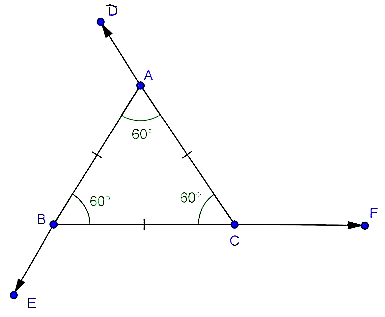$\mathrm{\angle }$ACF = $\mathrm{\angle }$ABC + $\mathrm{\angle }$BAC [$\because$ Exterior angle = sum of opposite interior angles]
$⇒$ $\mathrm{\angle }$ACF = 60o + 60o = 120o
Similarly, $\mathrm{\angle }$BAD = 120o and $\mathrm{\angle }$CBE = 120o

9. $\mathrm{\angle }$BAE = $\mathrm{\angle }$EDC = 52o (alternate angles)
$\therefore$ $\mathrm{\angle }$DEC = x = 180o - 40o - $\mathrm{\angle }$EDC  (because sum of all angles of a triangle is 180o)
= 180o - 40o - 52o
= 180o - 92o
= 88o

10. In $\mathrm{△}$ABD and $\mathrm{△}$ACD,
$\mathrm{△}$ABD$\cong$$\mathrm{△}$ACD = ...... [ASA axiom]
$\therefore$ AB = AC . . . .[c.p.c.t.]
$\therefore$ $\mathrm{△}$ABC is an isosceles triangle.

11. AB $‖$ DC and DB intersect them
$\mathrm{\angle }$BDC = $\mathrm{\angle }$DBA ...[Alternate angles]
In DPDC and D PBA
PD = PB ...[As P is the mid-point of BD]
$\mathrm{\angle }$PDC = $\mathrm{\angle }$PBA ...[As proved above]
$\mathrm{\angle }$DPC = $\mathrm{\angle }$BPA ...[Vertically opposite angles]
DPDC $\cong$ DPBA ...[By ASA property]
PC = PA ...[c.p.c.t.]
$⇒$ P is the mid-point of AC.

12.Given: AE = AD and BD = CE.
Proof: We have
AE = AD and CE = BD
$⇒$ AE + CE = AD + BD ..... (i)
$⇒$ AC = AB ...(ii)
Thus, Consider $\mathrm{△}AEB$ and $\mathrm{\Delta }$ADC, we have
$\mathrm{\angle }EAB=\mathrm{\angle }DAC$ [Common]
and, AC = AB [ From (ii)]
$\mathrm{△}AEB\cong \mathrm{△}ADC$ [ by SAS criterion ]
Hence proved

13. In $\mathrm{△}$ADE,
AD = AE . . . [Given]
∠AED = ∠ADE . . . .[∠s opposite to equal side of a $\mathrm{△}$ADE ]
180o – ∠AED = 180o – ∠ADE
In $\mathrm{△}$ADB and $\mathrm{△}$AEC,
AD = AE . . . [Given]
BD = EC . . . [Given]
∠ADB = ∠AEC . . . .[From (1)]
$\therefore \mathrm{△}ADB\cong \mathrm{△}AEC$ ........ [By SAS property]
$\therefore$ AB = AC ....... [c.p.c.t]

14. To Prove:

Construction: Take a point D on AC such that AD = AB. Join BD.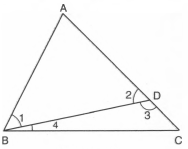Proof: In $\mathrm{△}$ABD, side AD has been produced to C.
$\therefore$ $\mathrm{\angle }$3 > $\mathrm{\angle }$1 [$\because$ Exterior angle of a $\mathrm{△}$ is greater than each of interior opp. angle] ...(i)
In $\mathrm{△}$BCD, side CD has been produced to A.
$\therefore$ $\mathrm{\angle }$2 > $\mathrm{\angle }$4 [$\because$ Exterior angle of a $\mathrm{△}$ is greater than each of interior opp. angle] ...(ii)
In $\mathrm{△}$ABD, we have
$⇒$ $\mathrm{\angle }$2 = $\mathrm{\angle }$1 [Angles opp. to equal sides are equal] ...(iii)
From (i) and (iii), we get
$\mathrm{\angle }$3 > $\mathrm{\angle }$2 ...(iv)
From (ii) and (iv), we get
$\mathrm{\angle }$3 > $\mathrm{\angle }$2 and $\mathrm{\angle }$2 > $\mathrm{\angle }$4
$⇒$ $\mathrm{\angle }$3 > $\mathrm{\angle }$4
$⇒$ BC > CD [Side opp to greater angle is larger]
$⇒$ CD < BC
$⇒$ AC - AD < BC
$⇒$ AC - AB < BC [$\because$ AD = AB]
Similarly, BC - AC < AB and BC - AB < AC

1. AC - AB < BC
2. BC - AC < AB
3. BC - AB < AC
15. Given: ABCD is a quadrilateral . AB = AD & CB = CD
To prove: AC is the perpendicular bisector of BD.
Proof: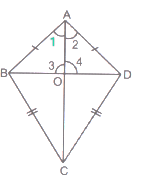Let diagonals AC & BD intersect at O.
Let, $\mathrm{\angle }BAC=\mathrm{\angle }1$$\mathrm{\angle }DAC=\mathrm{\angle }2$

In ∆ABC & ∆ADC, we have :-
BC = CD [Given]
AC = AC [Common side]
So, By SSS criterion of congruency of triangles , we have
$\mathrm{\Delta }ABC\cong \mathrm{\Delta }ADC$

$\therefore \mathrm{\angle }1=\mathrm{\angle }2$ [CPCT]

Now, in $\mathrm{\Delta }AOB$ and $\mathrm{\Delta }AOD$ , we have :-

$\mathrm{\angle }1=\mathrm{\angle }2$ [Proved above]

AO = AO [Common side]

So, By SAS criterion of congruency of triangles , we have :-

$\mathrm{\Delta }AOB\cong \mathrm{\Delta }AOD$

$\therefore \phantom{\rule{1em}{0ex}}BO=DO$ [CPCT]

And $\mathrm{\angle }3=\mathrm{\angle }4$ [CPCT]

But, $\mathrm{\angle }3+\mathrm{\angle }4={180}^{o}$ [Linear pair axiom]

$⇒\mathrm{\angle }3+\mathrm{\angle }3={180}^{\circ }\left[\because \mathrm{\angle }3=\mathrm{\angle }4\right]$

$⇒2\mathrm{\angle }3={180}^{\circ }$

$⇒\mathrm{\angle }3=\frac{{180}^{\circ }}{2}={90}^{\circ }$
$\therefore$ AC is perpendicular bisector of BD.  [$\because \mathrm{\angle }3={90}^{\circ }$ and BO = DO]
Hence, proved.